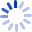# Journey Through Genius: The Great Theorems of Mathematics

Explores the "masterpieces" of mathematics, seventeen landmarks spanning 2,300 years and representing ten mathematicians. Each of these landmarks, to some degree, changed the way the world was perceived. Each theorem is presented with a description of the state of mathematics at the time, the development of the theorem, a biographical sketch of the mathematician and an outline of the proof with explanation.

##### Cash for Textbooks